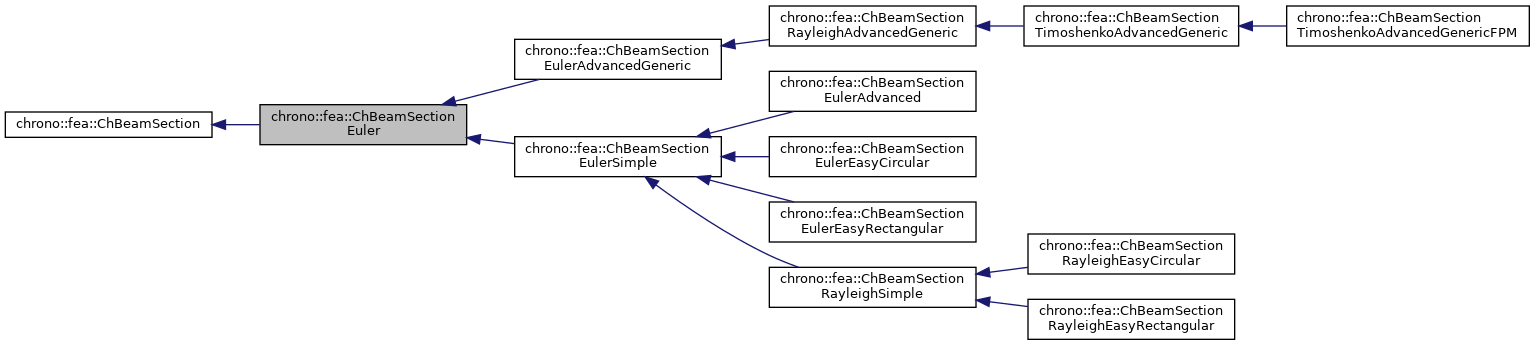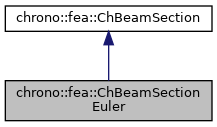chrono::fea::ChBeamSectionEuler Class Referenceabstract

## Description

Base class for all constitutive models of sections of Euler beams.

To be used with ChElementBeamEuler. For practical purposes, either you use the concrete inherited classes like ChBeamSectionEulerSimple, ChBeamSectionEulerAdvanced etc., or you inherit your class from this.

#include <ChBeamSectionEuler.h>

Inheritance diagram for chrono::fea::ChBeamSectionEuler:[legend]
Collaboration diagram for chrono::fea::ChBeamSectionEuler:[legend]

## Public Member Functions

virtual double GetAxialRigidity () const =0
Gets the axial rigidity, usually A*E, but might be ad hoc.

virtual double GetXtorsionRigidity () const =0
Gets the torsion rigidity, for torsion about X axis at elastic center, usually J*G, but might be ad hoc.

virtual double GetYbendingRigidity () const =0
Gets the bending rigidity, for bending about Y axis at elastic center, usually Iyy*E, but might be ad hoc.

virtual double GetZbendingRigidity () const =0
Gets the bending rigidity, for bending about Z axis at elastic center, usually Izz*E, but might be ad hoc.

virtual double GetSectionRotation () const =0
Set the rotation of the Y Z section axes for which the YbendingRigidity and ZbendingRigidity are defined.

virtual double GetCentroidY () const =0
Gets the Y position of the elastic center respect to centerline.

virtual double GetCentroidZ () const =0
Gets the Z position of the elastic center respect to centerline.

virtual double GetShearCenterY () const =0
Gets the Y position of the shear center respect to centerline.

virtual double GetShearCenterZ () const =0
Gets the Z position of the shear center respect to centerline.

virtual double GetMassPerUnitLength () const =0
Get mass per unit length, ex.SI units [kg/m].

virtual double GetInertiaJxxPerUnitLength () const =0
Get the Jxx component of the inertia per unit length (polar inertia) in the Y Z unrotated reference frame of the section at centerline. More...

virtual void ComputeInertiaMatrix (ChMatrixNM< double, 6, 6 > &M)=0
Compute the 6x6 sectional inertia matrix, as in {x_momentum,w_momentum}=[Mm]{xvel,wvel} The matrix is computed in the material reference (i.e. More...

virtual void ComputeInertiaDampingMatrix (ChMatrixNM< double, 6, 6 > &Ri, const ChVector<> &mW)
Compute the 6x6 sectional inertia damping matrix [Ri] (gyroscopic matrix damping), as in linearization dFi=[Mi]*d{xacc,wacc}+[Ri]*d{xvel,wvel}+[Ki]*d{pos,rot} The matrix is computed in the material reference, i.e. More...

virtual void ComputeInertiaStiffnessMatrix (ChMatrixNM< double, 6, 6 > &Ki, const ChVector<> &mWvel, const ChVector<> &mWacc, const ChVector<> &mXacc)
Compute the 6x6 sectional inertia stiffness matrix [Ki^], as in linearization dFi=[Mi]*d{xacc,wacc}+[Ri]*d{xvel,wvel}+[Ki]*d{pos,rot} The matrix is computed in the material reference. More...

virtual void ComputeQuadraticTerms (ChVector<> &mF, ChVector<> &mT, const ChVector<> &mW)=0
Compute the values of inertial force & torque depending on quadratic velocity terms, that is the gyroscopic torque (null for Euler beam as point-like mass section, might be nonzero if adding Rayleigh beam theory) and the centrifugal term (if any). More...

virtual void ComputeInertialForce (ChVector<> &mFi, ChVector<> &mTi, const ChVector<> &mWvel, const ChVector<> &mWacc, const ChVector<> &mXacc)
Compute the total inertial forces (per unit length). More...

void SetArtificialJyyJzzFactor (double mf)
The Euler beam model has no rotational inertia per each section, assuming mass is concentrated on the centerline. More...

double GetArtificialJyyJzzFactor ()

virtual void SetBeamRaleyghDampingAlpha (double malpha)
Set the "alpha" Rayleigh damping ratio,
the mass-proportional structural damping in: R = alpha*M + beta*K

double GetBeamRaleyghDampingAlpha ()

virtual void SetBeamRaleyghDampingBeta (double mbeta)
Set the "beta" Rayleigh damping ratio, the stiffness-proportional structural damping in: R = alpha*M + beta*K

double GetBeamRaleyghDampingBeta ()

virtual void SetBeamRaleyghDamping (double mbeta, double malpha=0)
Set both beta and alpha coefficients in Rayleigh damping model: R = alpha*M + beta*K. More...Public Member Functions inherited from chrono::fea::ChBeamSection
void SetDrawShape (std::shared_ptr< ChBeamSectionShape > mshape)
Set the graphical representation for this section. More...

std::shared_ptr< ChBeamSectionShapeGetDrawShape () const
Get the drawing shape of this section (i.e.a 2D profile used for drawing 3D tesselation and visualization) By default a thin square section, use SetDrawShape() to change it.

void SetDrawThickness (double thickness_y, double thickness_z)
Shortcut: adds a ChBeamSectionShapeRectangular for visualization as a centered rectangular beam, and sets its width/height. More...

Shortcut: adds a ChBeamSectionShapeCircular for visualization as a centered circular beam, and sets its radius. More...

void SetCircular (bool ic)
OBSOLETE only for backward compability

## Public Attributes

bool compute_inertia_damping_matrix = true
Flag that turns on/off the computation of the [Ri] 'gyroscopic' inertial damping matrix. More...

bool compute_inertia_stiffness_matrix = true
Flag that turns on/off the computation of the [Ki] inertial stiffness matrix. More...

bool compute_Ri_Ki_by_num_diff = false
Flag for computing the Ri and Ki matrices via numerical differentiation even if an analytical expression is provided. More...

## Protected Attributes

double rdamping_beta

double rdamping_alpha

double JzzJyy_factor

## Member Function Documentation

 void chrono::fea::ChBeamSectionEuler::ComputeInertiaDampingMatrix ( ChMatrixNM< double, 6, 6 > & Ri, const ChVector<> & mW )
virtual

Compute the 6x6 sectional inertia damping matrix [Ri] (gyroscopic matrix damping), as in linearization dFi=[Mi]*d{xacc,wacc}+[Ri]*d{xvel,wvel}+[Ki]*d{pos,rot} The matrix is computed in the material reference, i.e.

both linear and rotational coords assumed in the basis of the centerline reference. Default implementation: falls back to numerical differentiation of ComputeInertialForce to compute Ri, please override this if analytical formula of Ri is known!

Parameters
 Ri 6x6 sectional inertial-damping (gyroscopic damping) matrix values here mW current angular velocity of section, in material frame

## ◆ ComputeInertialForce()

 void chrono::fea::ChBeamSectionEuler::ComputeInertialForce ( ChVector<> & mFi, ChVector<> & mTi, const ChVector<> & mWvel, const ChVector<> & mWacc, const ChVector<> & mXacc )
virtual

Compute the total inertial forces (per unit length).

This default implementation falls back to Fi = [Mi]*{xacc,wacc}+{mF,mT} where [Mi] is given by ComputeInertiaMatrix() and {F_quad,T_quad} are given by ComputeQuadraticTerms(), i.e. gyro and centrif.terms. Note: both force and torque are returned in the basis of the material frame (not the absolute frame!), ex. to apply it to a Chrono body, the force must be rotated to absolute basis. For faster implementations one can override this, ex. avoid doing the [Mi] matrix product.

Parameters
 mFi total inertial force returned here, in basis of material frame mTi total inertial torque returned here, in basis of material frame mWvel current angular velocity of section, in material frame mWacc current angular acceleration of section, in material frame mXacc current acceleration of section, in material frame (not absolute!)

## ◆ ComputeInertiaMatrix()

 virtual void chrono::fea::ChBeamSectionEuler::ComputeInertiaMatrix ( ChMatrixNM< double, 6, 6 > & M )
pure virtual

Compute the 6x6 sectional inertia matrix, as in {x_momentum,w_momentum}=[Mm]{xvel,wvel} The matrix is computed in the material reference (i.e.

it is the sectional mass matrix)

Parameters
 M 6x6 sectional mass matrix values here

## ◆ ComputeInertiaStiffnessMatrix()

 void chrono::fea::ChBeamSectionEuler::ComputeInertiaStiffnessMatrix ( ChMatrixNM< double, 6, 6 > & Ki, const ChVector<> & mWvel, const ChVector<> & mWacc, const ChVector<> & mXacc )
virtual

Compute the 6x6 sectional inertia stiffness matrix [Ki^], as in linearization dFi=[Mi]*d{xacc,wacc}+[Ri]*d{xvel,wvel}+[Ki]*d{pos,rot} The matrix is computed in the material reference.

NOTE the matrix already contains the 'geometric' stiffness, so it transforms to absolute transl/local rot just like [Mi] and [Ri]: [Ki]_al =[R,0;0,I]*[Ki^]*[R',0;0,I'] , with [Ki^]=([Ki]+[0,f~';0,0]) for f=current force part of inertial forces. Default implementation: falls back to numerical differentiation of ComputeInertialForce to compute Ki^, please override this if analytical formula of Ki^ is known!

Parameters
 Ki 6x6 sectional inertial-stiffness matrix [Ki^] values here mWvel current angular velocity of section, in material frame mWacc current angular acceleration of section, in material frame mXacc current acceleration of section, in material frame (not absolute!)

 virtual void chrono::fea::ChBeamSectionEuler::ComputeQuadraticTerms ( ChVector<> & mF, ChVector<> & mT, const ChVector<> & mW )
pure virtual

Compute the values of inertial force & torque depending on quadratic velocity terms, that is the gyroscopic torque (null for Euler beam as point-like mass section, might be nonzero if adding Rayleigh beam theory) and the centrifugal term (if any).

All terms expressed in the material reference, ie. the reference in the centerline of the section.

Parameters
 mF centrifugal term (if any) returned here mT gyroscopic term returned here mW current angular velocity of section, in material frame

## ◆ GetInertiaJxxPerUnitLength()

 virtual double chrono::fea::ChBeamSectionEuler::GetInertiaJxxPerUnitLength ( ) const
pure virtual

Get the Jxx component of the inertia per unit length (polar inertia) in the Y Z unrotated reference frame of the section at centerline.

Note: it automatically follows Jxx=Jyy+Jzz for the polar theorem. Also, Jxx=density*Ixx if constant density.

## ◆ SetArtificialJyyJzzFactor()

 void chrono::fea::ChBeamSectionEuler::SetArtificialJyyJzzFactor ( double mf )
inline

The Euler beam model has no rotational inertia per each section, assuming mass is concentrated on the centerline.

However this creates a singular mass matrix, that might end in problems when doing modal analysis etc. A solution is to force Jyy and Jzz inertials per unit lengths to be a percent of the mass per unit length. By default it is 1/500. Use this function to set such factor. You can also turn it to zero. Note that the effect becomes negligible anyway for finer meshing.

## ◆ SetBeamRaleyghDamping()

 virtual void chrono::fea::ChBeamSectionEuler::SetBeamRaleyghDamping ( double mbeta, double malpha = 0 )
inlinevirtual

Set both beta and alpha coefficients in Rayleigh damping model: R = alpha*M + beta*K.

For backward compatibility, if one provides only the first parameter, this would be the "beta" stiffness-proportional term, and the "alpha" mass proportional term would be left to default zero.

## ◆ compute_inertia_damping_matrix

 bool chrono::fea::ChBeamSectionEuler::compute_inertia_damping_matrix = true

Flag that turns on/off the computation of the [Ri] 'gyroscopic' inertial damping matrix.

If false, Ri=0. Can be used for cpu speedup, profiling, tests. Default: true.

## ◆ compute_inertia_stiffness_matrix

 bool chrono::fea::ChBeamSectionEuler::compute_inertia_stiffness_matrix = true

Flag that turns on/off the computation of the [Ki] inertial stiffness matrix.

If false, Ki=0. Can be used for cpu speedup, profiling, tests. Default: true.

## ◆ compute_Ri_Ki_by_num_diff

 bool chrono::fea::ChBeamSectionEuler::compute_Ri_Ki_by_num_diff = false

Flag for computing the Ri and Ki matrices via numerical differentiation even if an analytical expression is provided.

Children calsses must take care of this. Default: false.

The documentation for this class was generated from the following files:
• /builds/uwsbel/chrono/src/chrono/fea/ChBeamSectionEuler.h
• /builds/uwsbel/chrono/src/chrono/fea/ChBeamSectionEuler.cpp